## 4.14.5. Distributed Array Datatype Constructor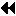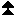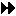Up: New Datatype Manipulation Functions Next: New Predefined Datatypes Previous: Subarray Datatype Constructor

The distributed array type constructor supports HPF-like  data distributions. However, unlike in HPF, the storage order may be specified for C arrays as well as for Fortran arrays.

One can create an HPF-like file view using this type constructor as follows. Complementary filetypes are created by having every process of a group call this constructor with identical arguments (with the exception of rank which should be set appropriately). These filetypes (along with identical disp and etype) are then used to define the view (via MPI_FILE_SET_VIEW). Using this view, a collective data access operation (with identical offsets) will yield an HPF-like distribution pattern. ( End of advice to users.)
MPI_TYPE_CREATE_DARRAY(size, rank, ndims, array_of_gsizes, array_of_distribs, array_of_dargs, array_of_psizes, order, oldtype, newtype)
[ IN size] size of process group (positive integer)
[ IN rank] rank in process group (nonnegative integer)
[ IN ndims] number of array dimensions as well as process grid dimensions (positive integer)
[ IN array_of_gsizes] number of elements of type oldtype in each dimension of global array (array of positive integers)
[ IN array_of_distribs] distribution of array in each dimension (array of state)
[ IN array_of_dargs] distribution argument in each dimension (array of positive integers)
[ IN array_of_psizes] size of process grid in each dimension (array of positive integers)
[ IN order] array storage order flag (state)
[ IN oldtype] old datatype (handle)
[ OUT newtype] new datatype (handle)

int MPI_Type_create_darray(int size, int rank, int ndims, int array_of_gsizes[], int array_of_distribs[], int array_of_dargs[], int array_of_psizes[], int order, MPI_Datatype oldtype, MPI_Datatype *newtype)
MPI_TYPE_CREATE_DARRAY(SIZE, RANK, NDIMS, ARRAY_OF_GSIZES, ARRAY_OF_DISTRIBS, ARRAY_OF_DARGS, ARRAY_OF_PSIZES, ORDER, OLDTYPE, NEWTYPE, IERROR)
INTEGER SIZE, RANK, NDIMS, ARRAY_OF_GSIZES(*), ARRAY_OF_DISTRIBS(*), ARRAY_OF_DARGS(*), ARRAY_OF_PSIZES(*), ORDER, OLDTYPE, NEWTYPE, IERROR
MPI::Datatype MPI::Datatype::Create_darray(int size, int rank, int ndims, const int array_of_gsizes[], const int array_of_distribs[], const int array_of_dargs[], const int array_of_psizes[], int order) const

MPI_TYPE_CREATE_DARRAY can be used to generate the datatypes corresponding to the distribution of an ndims-dimensional array of oldtype elements onto an ndims-dimensional grid of logical processes. Unused dimensions of array_of_psizes should be set to 1. (See Example Distributed Array Datatype Constructor .) For a call to MPI_TYPE_CREATE_DARRAY to be correct, the equation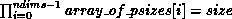must be satisfied. The ordering of processes in the process grid is assumed to be row-major, as in the case of virtual Cartesian process topologies in MPI-1.

For both Fortran and C arrays, the ordering of processes in the process grid is assumed to be row-major. This is consistent with the ordering used in virtual Cartesian process topologies in MPI-1. To create such virtual process topologies, or to find the coordinates of a process in the process grid, etc., users may use the corresponding functions provided in MPI-1. ( End of advice to users.)

Each dimension of the array can be distributed in one of three ways:

• MPI_DISTRIBUTE_BLOCK - Block distribution
• MPI_DISTRIBUTE_CYCLIC - Cyclic distribution
• MPI_DISTRIBUTE_NONE - Dimension not distributed.

The constant MPI_DISTRIBUTE_DFLT_DARG specifies a default distribution argument. The distribution argument for a dimension that is not distributed is ignored. For any dimension i in which the distribution is MPI_DISTRIBUTE_BLOCK, it erroneous to specify array_of_dargs[i] * array_of_psizes[i] < array_of_gsizes[i].

For example, the HPF layout ARRAY(CYCLIC(15)) corresponds to MPI_DISTRIBUTE_CYCLIC with a distribution argument of 15, and the HPF layout ARRAY(BLOCK) corresponds to MPI_DISTRIBUTE_BLOCK with a distribution argument of MPI_DISTRIBUTE_DFLT_DARG.

The order argument is used as in MPI_TYPE_CREATE_SUBARRAY to specify the storage order. Therefore, arrays described by this type constructor may be stored in Fortran (column-major) or C (row-major) order. Valid values for order are MPI_ORDER_FORTRAN and MPI_ORDER_C.

This routine creates a new MPI datatype with a typemap defined in terms of a function called ``cyclic()'' (see below).

Without loss of generality, it suffices to define the typemap for the MPI_DISTRIBUTE_CYCLIC case where MPI_DISTRIBUTE_DFLT_DARG is not used.

MPI_DISTRIBUTE_BLOCK and MPI_DISTRIBUTE_NONE can be reduced to the MPI_DISTRIBUTE_CYCLIC case for dimension i as follows.

MPI_DISTRIBUTE_BLOCK with array_of_dargs[i] equal to MPI_DISTRIBUTE_DFLT_DARG is equivalent to MPI_DISTRIBUTE_CYCLIC with array_of_dargs[i] set to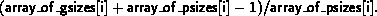If array_of_dargs[i] is not MPI_DISTRIBUTE_DFLT_DARG, then MPI_DISTRIBUTE_BLOCK and MPI_DISTRIBUTE_CYCLIC are equivalent.

MPI_DISTRIBUTE_NONE is equivalent to MPI_DISTRIBUTE_CYCLIC with array_of_dargs[i] set to array_of_gsizes[i].

Finally, MPI_DISTRIBUTE_CYCLIC with array_of_dargs[i] equal to MPI_DISTRIBUTE_DFLT_DARG is equivalent to MPI_DISTRIBUTE_CYCLIC with array_of_dargs[i] set to 1.

For MPI_ORDER_FORTRAN, an ndims-dimensional distributed array ( newtype) is defined by the following code fragment:

```oldtype = oldtype;
for ( i = 0; i < ndims; i++ ) {
oldtype[i+1] = cyclic(array_of_dargs[i],
array_of_gsizes[i],
r[i],
array_of_psizes[i],
oldtype[i]);
}
newtype = oldtype[ndims];
```
For MPI_ORDER_C, the code is:

```oldtype = oldtype;
for ( i = 0; i < ndims; i++ ) {
oldtype[i + 1] = cyclic(array_of_dargs[ndims - i - 1],
array_of_gsizes[ndims - i - 1],
r[ndims - i - 1],
array_of_psizes[ndims - i - 1],
oldtype[i]);
}
newtype = oldtype[ndims];

```
where r[i] is the position of the process (with rank rank) in the process grid at dimension i. The values of r[i] are given by the following code fragment:

```t_rank = rank;
t_size = 1;
for (i = 0; i < ndims; i++)
t_size *= array_of_psizes[i];
for (i = 0; i < ndims; i++) {
t_size = t_size / array_of_psizes[i];
r[i] = t_rank / t_size;
t_rank = t_rank % t_size;
}
```
Let the typemap of oldtype have the form: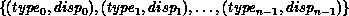where typei is a predefined MPI datatype, and let ex be the extent of oldtype.

Given the above, the function cyclic() is defined as follows: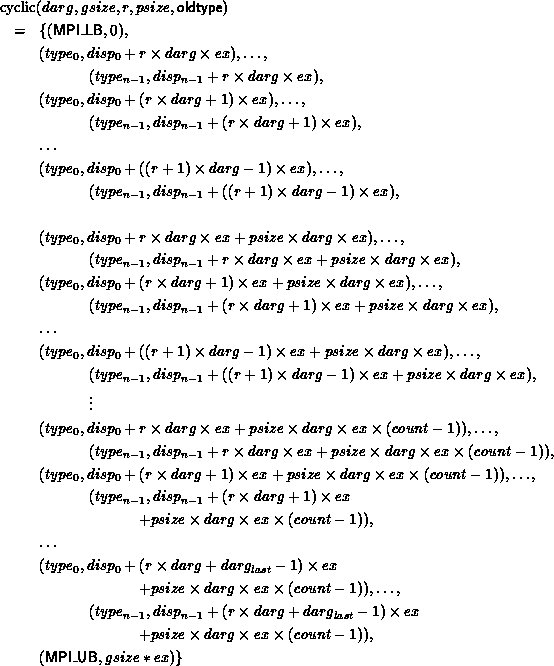where count is defined by this code fragment:

```nblocks = (gsize + (darg - 1)) / darg;
count = nblocks / psize;
left_over = nblocks - count * psize;
if (r < left_over)
count = count + 1;
```
Here, nblocks is the number of blocks that must be distributed among the processors. Finally, darglast is defined by this code fragment:
```if ((num_in_last_cyclic = gsize % (psize * darg)) == 0)
darg_last = darg;
else
darg_last = num_in_last_cyclic - darg * r;
if (darg_last > darg)
darg_last = darg;
if (darg_last <= 0)
darg_last = darg;
```

Example Consider generating the filetypes corresponding to the HPF distribution:

```<oldtype> FILEARRAY(100, 200, 300)
!HPF\$ PROCESSORS PROCESSES(2, 3)
!HPF\$ DISTRIBUTE FILEARRAY(CYCLIC(10), *, BLOCK) ONTO PROCESSES
```
This can be achieved by the following Fortran code, assuming there will be six processes attached to the run:
```ndims = 3
array_of_gsizes(1) = 100
array_of_distribs(1) = MPI_DISTRIBUTE_CYCLIC
array_of_dargs(1) = 10
array_of_gsizes(2) = 200
array_of_distribs(2) = MPI_DISTRIBUTE_NONE
array_of_dargs(2) = 0
array_of_gsizes(3) = 300
array_of_distribs(3) = MPI_DISTRIBUTE_BLOCK
array_of_dargs(3) = MPI_DISTRIBUTE_DFLT_ARG
array_of_psizes(1) = 2
array_of_psizes(2) = 1
array_of_psizes(3) = 3
call MPI_COMM_SIZE(MPI_COMM_WORLD, size, ierr)
call MPI_COMM_RANK(MPI_COMM_WORLD, rank, ierr)
call MPI_TYPE_CREATE_DARRAY(size, rank, ndims, array_of_gsizes, &
array_of_distribs, array_of_dargs, array_of_psizes,        &
MPI_ORDER_FORTRAN, oldtype, newtype, ierr)
```Up: New Datatype Manipulation Functions Next: New Predefined Datatypes Previous: Subarray Datatype Constructor